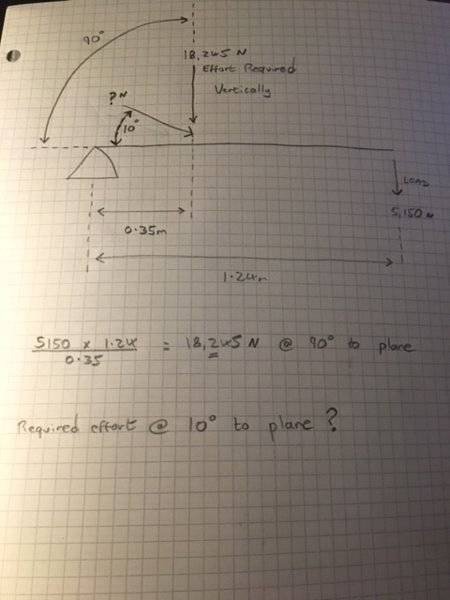# Class 3 Lever: Non-perpendicular Effort

Jimbo86
Hi,

We have a machine in our workshop in which we are modifying to relieve the amount of lifting force required by the hydraulic ram. This will subsequently reduce hydraulic system pressure which is the main goal. I'm trying to calculate the current configuration. It consists of a class 3 lever, with the effort applied at a 30 degree angle to the horizontal plane.I've tried the following equation:

5150 * 1.24 * sin(10) / 0.35 = 3168N

I know the above is incorrect. At sin(90) perpendicular effort I need 18,245 N to lift the load. I would expect an answer of 3 to 4 times this with the effort applied at 10 degrees.

Please can someone point me in the right direction.

Many thanks

#### Attachments

Homework Helper
Gold Member
Hint..

N*Sin(10) must equal 18245

Rearrange to give N.

Jimbo86

((5150 * 1.24) / sin(10)) / 0.35

This gives me 105,072.9 newtons which equals 10.71 tonne.

Have I hit it?

Homework Helper
Gold Member
Yes.

Although I'd work slightly differently it as...

Vertical component (Nv) of N =
(5150*1.24)/0.35 = 18245

Then
Nv = N Sin(10)
so
N = Nv/sin(10)
= 18245/sin(10)
= 105,068 Newtons

Jimbo86
Excellent.